1 Draw the graph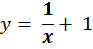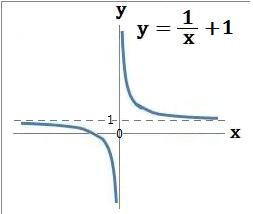2 Draw the graph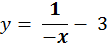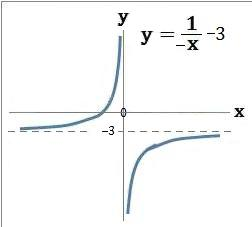3 Draw the graph y = x3 + 274 Draw the graph y = −x3 − 64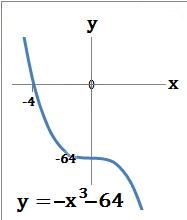5 Draw the graph y = 2x + 3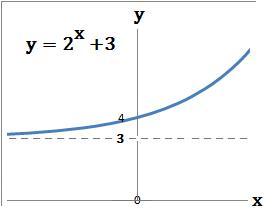6 Draw the graph y= 2−x − 2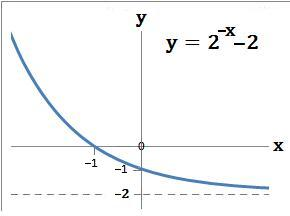back to: